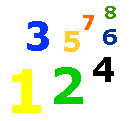Addition Facts - Basic Facts - Elementary Math Lessons & Tests - My Schoolhouse - Online Learning

Complete the basic facts (0-9) addition problems.

 7 + 2
 5 + 1
 8 + 2
 8 + 8
 0 + 2
 7 + 0
 1 + 1
 6 + 3
 1 + 4
 2 + 00 + 3
 6 + 7
 3 + 2
 6 + 9
 1 + 0
 5 + 4
 6 + 0
 9 + 5
 3 + 2
 5 + 23 + 1
 9 + 8
 0 + 4
 3 + 3
 9 + 4
 2 + 5
 3 + 7
 0 + 9
 3 + 5
 1 + 31 + 7
 6 + 1
 2 + 1
 3 + 0
 9 + 9
 2 + 1
 4 + 5
 2 + 6
 8 + 0
 4 + 19 + 1
 3 + 2
 5 + 0
 4 + 5
 8 + 0
 3 + 8
 3 + 4
 0 + 9
 7 + 5
 5 + 56 + 3
 7 + 4
 9 + 5
 8 + 9
 0 + 1
 1 + 6
 6 + 6
 2 + 1
 2 + 8
 4 + 23 +7
 6 + 5
 1 + 5
 0 + 8
 9 + 1
 6 + 8
 9 + 7
 2 + 7
 5 + 7
 0 + 04 + 6
 0 + 8
 6 + 8
 2 + 9
 7 + 7
 9 + 1
 7 + 3
 1 + 5
 4 + 3
 2 + 47 + 0
 9 + 3
 7 + 4
 8 + 1
 3 + 0
 1 + 4
 2 + 7
 2 + 2
 3 + 2
 7 + 97 + 7
 2 + 9
 5 + 7
 4 + 8
 5 + 8
 9 + 6
 6 + 5
 4 + 2
 4 + 9
 6 + 8